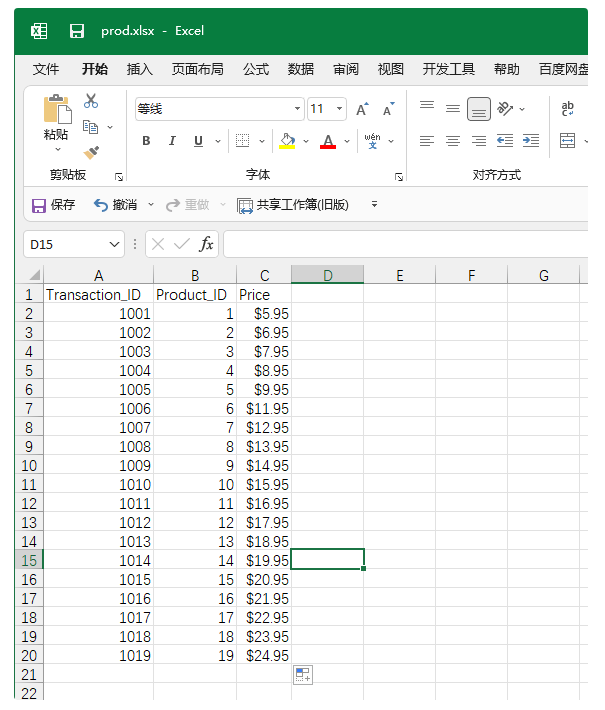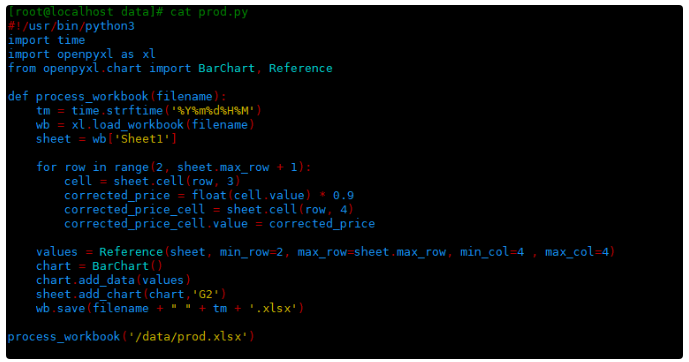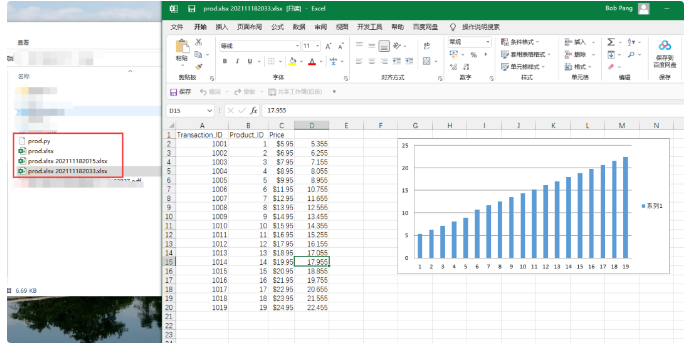2023
05-25

# python表格处理教程（Python 自动化处理 Excel 表格）# yum -y install python3-openpyxl

[root@localhost data]# vim prod.py
#!/usr/bin/python3
import time
import openpyxl as xl
from openpyxl.chart import BarChart, Reference
def process_workbook(filename):
tm = time.strftime('%Y%m%d%H%M')
sheet = wb['Sheet1']
for row in range(2, sheet.max_row + 1):
cell = sheet.cell(row, 3)
corrected_price = float(cell.value) * 0.9
corrected_price_cell = sheet.cell(row, 4)
corrected_price_cell.value = corrected_price
values = Reference(sheet, min_row=2, max_row=sheet.max_row, min_col=4 , max_col=4)
chart = BarChart()
wb.save(filename + " " + tm + '.xlsx')
process_workbook('/data/prod.xlsx')import time
import openpyxl as xl
from openpyxl.chart import BarChart, Reference

def process_workbook(filename):

tm = time.strftime('%Y%m%d%H%M')
sheet = wb['Sheet1']

for row in range(2, sheet.max_row + 1):
cell = sheet.cell(row,3)

corrected_price = float(cell.value) * 0.9
corrected_price_cell = sheet.cell(row, 4)
corrected_price_cell.value = corrected_price

values = Reference(sheet, min_row=2, max_row=sheet.max_row, min_col=4 , max_col=4)

chart = BarChart()

wb.save(filename + " " + tm + '.xlsx')

process_workbook('/data/prod.xlsx')

[root@localhost data]# chmod +x /data/prod.py
[root@localhost data]# /data/prod.py